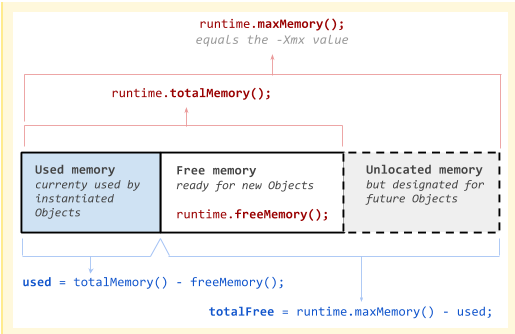# Android Code SnippetMemory Usage

#### Robert Valentino

##### Well-Known Member
Of my 235+ users I have one (using a Samsung S6) that is generating out of memory very often.

This code hasn't helped - I never seem to use more than what is shown yet their device seems to get the out of memory

Maybe this will help someone else

Bottom of articleB4X:
``````Public  Sub MemoryUsage As String
Dim r            As Reflector
Dim Text        As String

Dim MaxMemory    As Long
Dim FreeMemory   As Long
Dim TotalMemory As Long
Dim UsedMemory   As Long
Dim TotalFree   As Long

r.Target    = r.RunStaticMethod("java.lang.Runtime", "getRuntime", Null, Null)

MaxMemory      = r.RunMethod("maxMemory")
FreeMemory    = r.RunMethod("freeMemory")
TotalMemory   = r.RunMethod("totalMemory")
UsedMemory   = TotalMemory - FreeMemory
TotalFree   = MaxMemory   - UsedMemory

Text = "  Max Memory = " &NumberFormat2((MaxMemory/(1024*1024)), 1, 2, 2, True) &" MB"                        _
&CRLF &" Total Free        = " &NumberFormat2((TotalFree/(1024*1024)), 1, 2, 2, True) &" MB"       _
&CRLF &" Total Memory = " &NumberFormat2((TotalMemory/(1024*1024)), 1, 2, 2, True) &" MB"            _
&CRLF &" Used Memory = " &NumberFormat2((UsedMemory/(1024*1024)), 1, 2, 2, True) &" MB"               _
&CRLF &"  Free Memory = " &NumberFormat2((FreeMemory/(1024*1024)), 1, 2, 2, True) &" MB"

Log("  Max Memory = " & NumberFormat2((MaxMemory   / (1024*1024)), 1, 2, 2, True) & " MB")
Log("Total Free   = " & NumberFormat2((UsedMemory      / (1024*1024)), 1, 2, 2, True) & " MB")
Log("Total Memory = " & NumberFormat2((TotalMemory    / (1024*1024)), 1, 2, 2, True) & " MB")
Log(" Used Memory = " & NumberFormat2((UsedMemory      / (1024*1024)), 1, 2, 2, True) & " MB")
Log(" Free Memory = " & NumberFormat2((FreeMemory      / (1024*1024)), 1, 2, 2, True) & " MB")

Return Text
End Sub``````
Displays a Dialog and Log Messages

Log:
Max Memory = 512.00 MB
Total Free = 128.98 MB
Total Memory = 139.61 MB
Used Memory = 128.98 MB
Free Memory = 10.63 MB

Dialog:#### KMatle

##### Expert
512 MBWhat device is it?